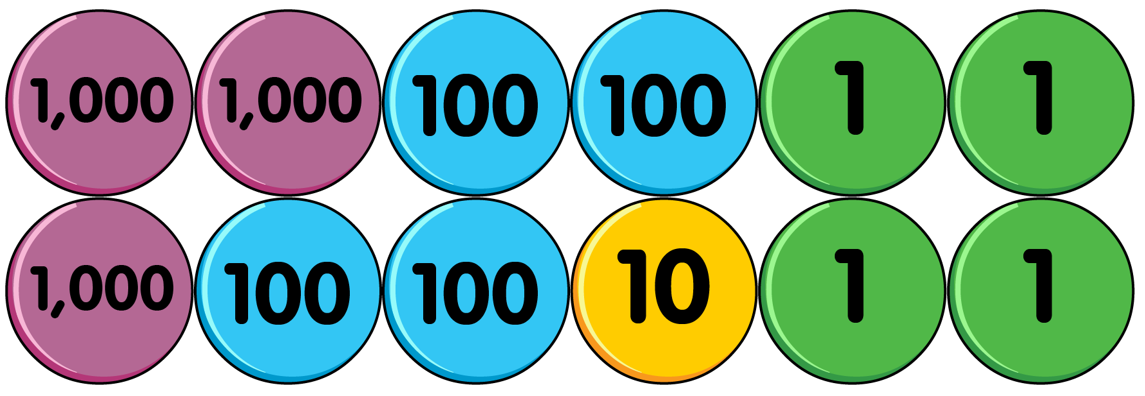# October 5th

## 5th October 2023

##### Add and Subtract Ones, Tens, Hundreds and Thousands

• Which number is represented by the counters?• Add 2 thousands to the number represented in question 1.
• Subtract 2 ones from the answer of question 4.
• Subtract 5 tens from the answer to question 5.

• Correct any incorrect answers. a) 2,483 + 300 = 5,483     b) 4,322 - 400 = 4,922     c) 3,816 + 2,000 = 1,816

• 3,414
• 5,414
• 5,914
• 5,944
• 5,942
• 5,892